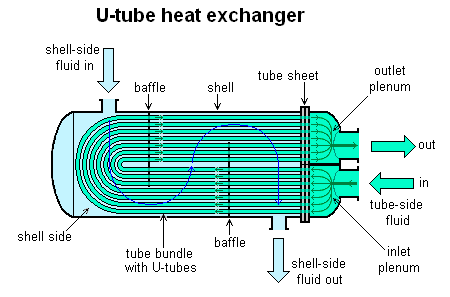# Shell & Tube Heat Exchanger Sizing Calculator for shellside

This is a calculator for sizing a shell and tube heat exchanger with tubeside flow fixed.

With shellside and tubeside inlet/outlet temperatures fixed, the required shellside flow is calculated corresponding to given tubeside flow. The log mean temperature difference (LMTD) is also reported.For known shellside and tubeside dimensions heat transfer areas and heat transfer coefficients on both sides are calculated.

Finally the overall heat transfer coefficient is calculated and reported. If a different value of overall heat transfer coefficient is assumed or desired, then that value should be specified in the end section.

The required heat transfer area corresponding to the assumed overall heat transfer coefficient is then reported.

 Shellside inlet T 0C Shellside outlet T 0C Tubeside inlet T 0C Tubeside outlet T 0C Tubeside mass flow kg/hr Shellside fluid density kg/m3 Tubeside fluid density kg/m3 Shellside fluid viscosity cP Tubeside fluid viscosity cP Shellside specific heat Kcal/kg.0C Tubeside specific heat Kcal/kg.0C Shellside conductivity Btu/hr.ft.0F Tubeside conductivity Btu/hr.ft.0F LMTD correction factor (Ft) Tubeside Dimensions Tube I.D. inches Tube O.D. inches Tube Length feet Number of tubes Tube pitch inches Number of tube passes Total tube area (at) inch2 Shellside Dimensions Shell Diameter inches Baffle Spacing inches Shellside equivalent diameter (De) inches Number of shell passes LMTD 0C Heat Exchanged Kcal/hr Corresponding Shellside Flow kg/hr Shellside Flow Area ft2 Tubeside Flow Area ft2 Shellside Heat Transfer Coefficient Btu/hr.ft2F Tubeside Heat Transfer Coefficient Btu/hr.ft2F Calculated overall heat transfer coefficient Btu/hr.ft2F Assumed overall heat transfer coefficient Btu/hr.ft2F Area required based on assumed heat transfer coefficient m2

## Heat exchanger calculators

Note all of the following calculators are for demo. To access the actual working calculators, you will need to create a login on EnggCyclopedia.

1. This quick LMTD calculator helps to quickly get LMTD value for an exchanger.
2. Then there is another calculator for LMTD correction factor.
3. Apart from these, you will also need to calculate the pressure drop on the shell and tube sides for your process datasheet. This calculator is for shell side pressure drop calculation.
4. You can use this calculator to determine tubeside pressure drop.
5. Finally there's another heat exchanger area calculator for fixed shell side flow to help you with sizing calculations on the tubeside.

Apart from these calculators, you can always use a heat exchanger design software to build a model of your heat exchanger design and then to simulate its performance.

## Tutorials for shell & tube exchanger calculations

Here are some step by step guided tutorials about how to use those calculators for shell & tube heat exchanger calculations. In these tutorials, we will make use of the shell & tube heat exchanger equations discussed above.